Next: 3.4 Miller Index Notation Up: 3. Strain and Semiconductor Previous: 3.2 Stress

# 3.3 Stress-Strain Relation

Within the elastic limit of a material Hooke's law is a good approximation for relating stress and strain The generalization of Hook's law for three-dimensional elastic bodies leads to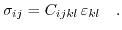(3.10)

The elastic stiffness tensor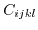is of fourth order and contains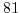(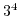) elements. Introducing additional symmetry considerations, the number of needed components can be reduced . Cubic semiconductors like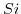,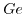or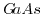are characterized by only three constants (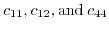).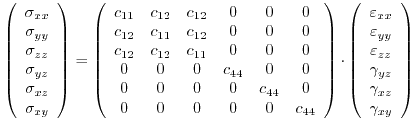(3.11)

If the strain, instead of stress, is the quantity of interest, it can be calculated by inversion of the elastic stiffness tensor (3.10)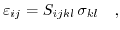(3.12)

or in matrix form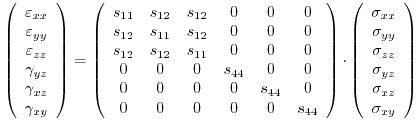(3.13)

The stiffness constants are normally denoted as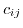, while the compliance constants are named as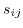. The compliance constants can be calculated from the stiffness constants with the following relations: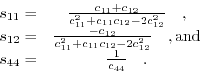(3.14)Next: 3.4 Miller Index Notation Up: 3. Strain and Semiconductor Previous: 3.2 Stress

T. Windbacher: Engineering Gate Stacks for Field-Effect Transistors